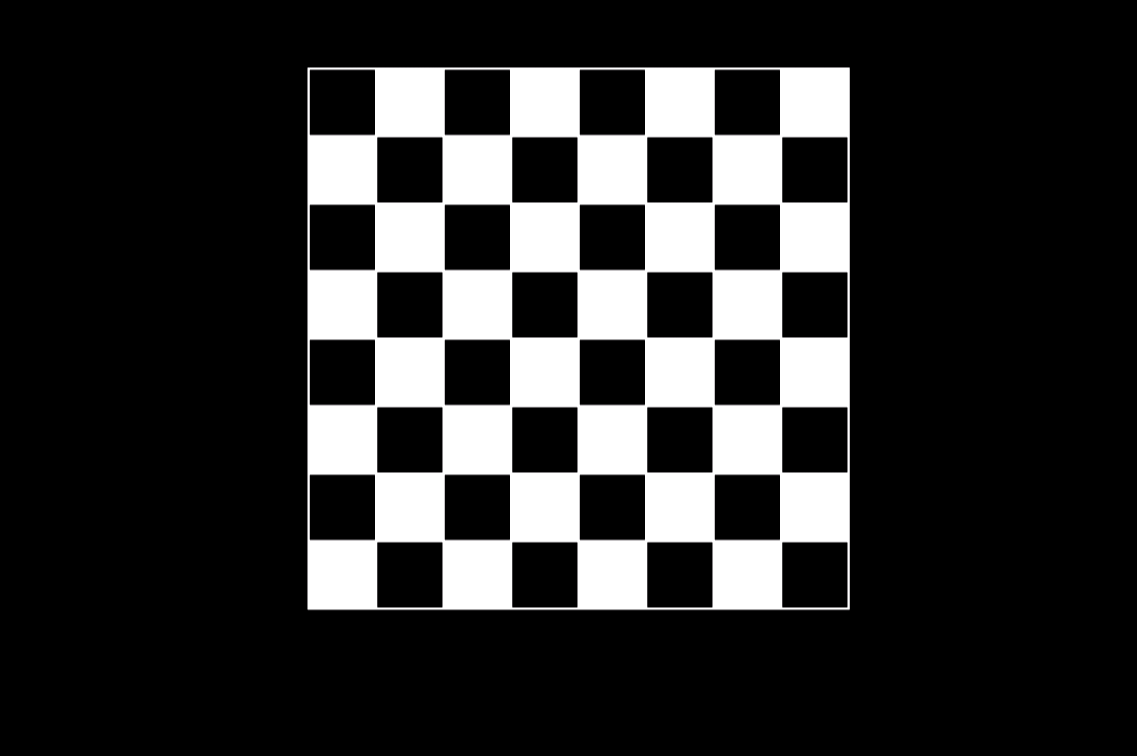# Draw a Chess Board using Graphics Programming in C

• Last Updated : 21 Jun, 2020

Prerequisite: graphics.h, How to include graphics.h in CodeBlocks?

In Computer Graphics, we use graphics.h which provide direct functions to draw different coordinate shapes(like circle, rectangle etc). By using these functions we can draw different objects like car, hut, trees etc. In this program, the task is to draw a Chess Board using the functions in graphics.

To run the program we have the include the below header file:

```#include <graphic.h>
```

Approach: We will create a Chess Board with the help below functions:

1. rectangle(left, top, right, bottom): A function from graphics.h header file which is used to draw a rectangle. Coordinates of the left top and right bottom corners are required to draw the rectangle. left specifies the X-coordinate of the top left corner, top specifies the Y-coordinate of the top left corner, right specifies the X-coordinate of the right bottom corner, bottom specifies the Y-coordinate of the right bottom corner.
2. delay(): This function is present in library “dos.h” is used for holding the program output for a small period of time since processing is very fast so use it to see the result.
3. setcolor(): A function from graphics.h header file which sets the color of the pointer (cursor). There are some predefined colors in computer graphics. Here n is the color number.
4. setfillstyle(): A function from graphics.h header file which sets the current fill pattern and fill color.
5. floodfill(): A function from graphics.h header file which is used to fill an enclosed area.

Below is the implementation of to draw Chess Board using graphics in C:

 `// C program to create a chess board``#include ``#include ``#include ``#include `` ` `// Driver Code``void` `main()``{``    ``// Auto detection``    ``int` `gr = DETECT, gm;``    ``int` `r, c, x = 30, y = 30, black = 0;`` ` `    ``// Initialize graphics mode by passing``    ``// three arguments to initgraph function`` ` `    ``// &gdriver is the address of gdriver``    ``// variable, &gmode is the address of``    ``// gmode and  "C:\\Turboc3\\BGI" is the``    ``// directory path where BGI files are stored``    ``initgraph(&gr, &gm, ``"C:\\TURBOC3\\BGI"``);`` ` `    ``// Iterate over 8 rows``    ``for` `(r = 0; r < 8; r++) {`` ` `        ``// iterate over 8 cols``        ``for` `(c = 1; c <= 8; c++) {`` ` `            ``// If current block is to``            ``// color as black``            ``if` `(black == 0) {`` ` `                ``// set next color as white``                ``setcolor(WHITE);`` ` `                ``// sets the current fill``                ``// pattern and fill color``                ``// for black boxes``                ``setfillstyle(SOLID_FILL, BLACK);`` ` `                ``// creating rectangle``                ``// with length and breadth``                ``// with size 30``                ``rectangle(x, y, x + 30, y + 30);`` ` `                ``// Fill an enclosed area``                ``floodfill(x + 1, y + 1, WHITE);`` ` `                ``// Set black to true``                ``black = 1;``            ``}`` ` `            ``// If current block is to``            ``// color as white``            ``else` `{``                ``setcolor(WHITE);`` ` `                ``// sets the current fill``                ``// pattern and fill color``                ``// for whitw boxes``                ``setfillstyle(SOLID_FILL, WHITE);`` ` `                ``// creating rectangle``                ``// with length and breadth``                ``// with size 30``                ``rectangle(x, y, x + 30, y + 30);`` ` `                ``// Fill an enclosed area``                ``floodfill(x + 1, y + 1, WHITE);`` ` `                ``// Set black to false``                ``black = 0;``            ``}`` ` `            ``// Increment for next row``            ``x = x + 30;`` ` `            ``// delay function under library``            ``// "dos.h" for holding the``            ``// function for some time``            ``delay(30);``        ``}``        ``if` `(black == 0)``            ``black = 1;``        ``else``            ``black = 0;`` ` `        ``delay(30);``        ``x = 30;``        ``y = 30 + y;``    ``}`` ` `    ``// getch is used to hold the output screen``    ``// and wait until user gives any type of``    ``// input in turbo c``    ``getch();`` ` `    ``// close graph function is used to exit``    ``// from the graphics screen``    ``closegraph();``}`

Output:
Below is the output of the above program:My Personal Notes arrow_drop_up